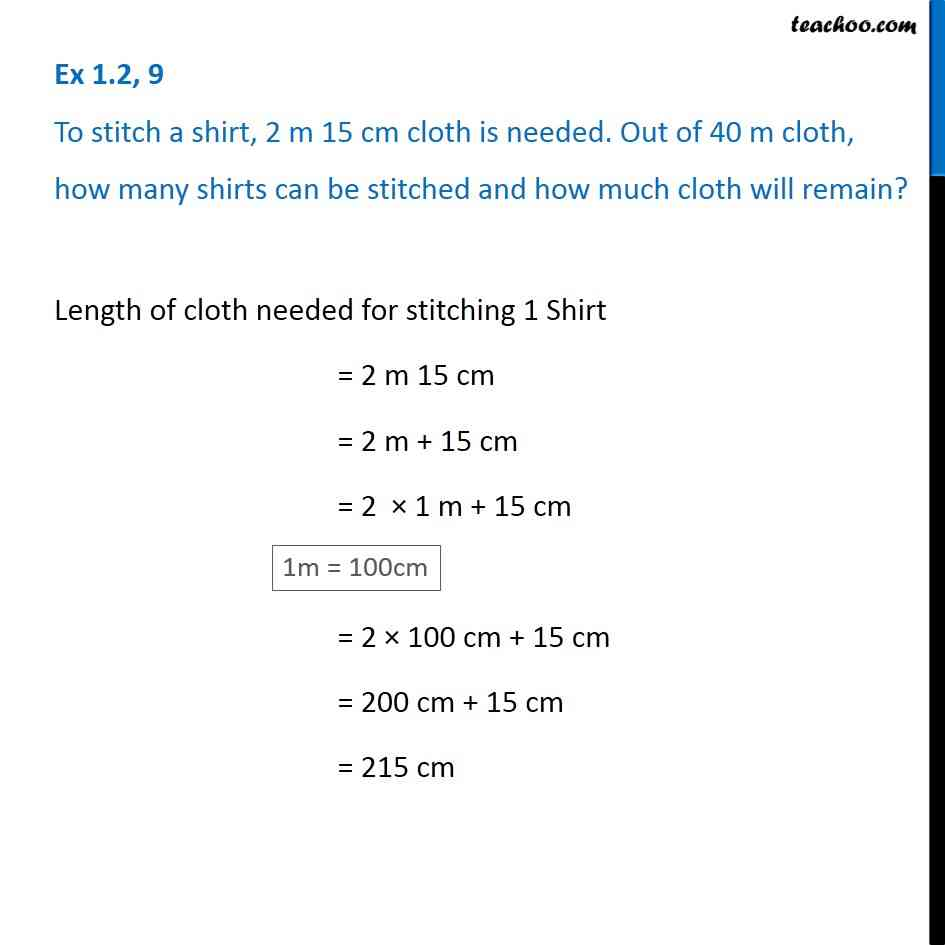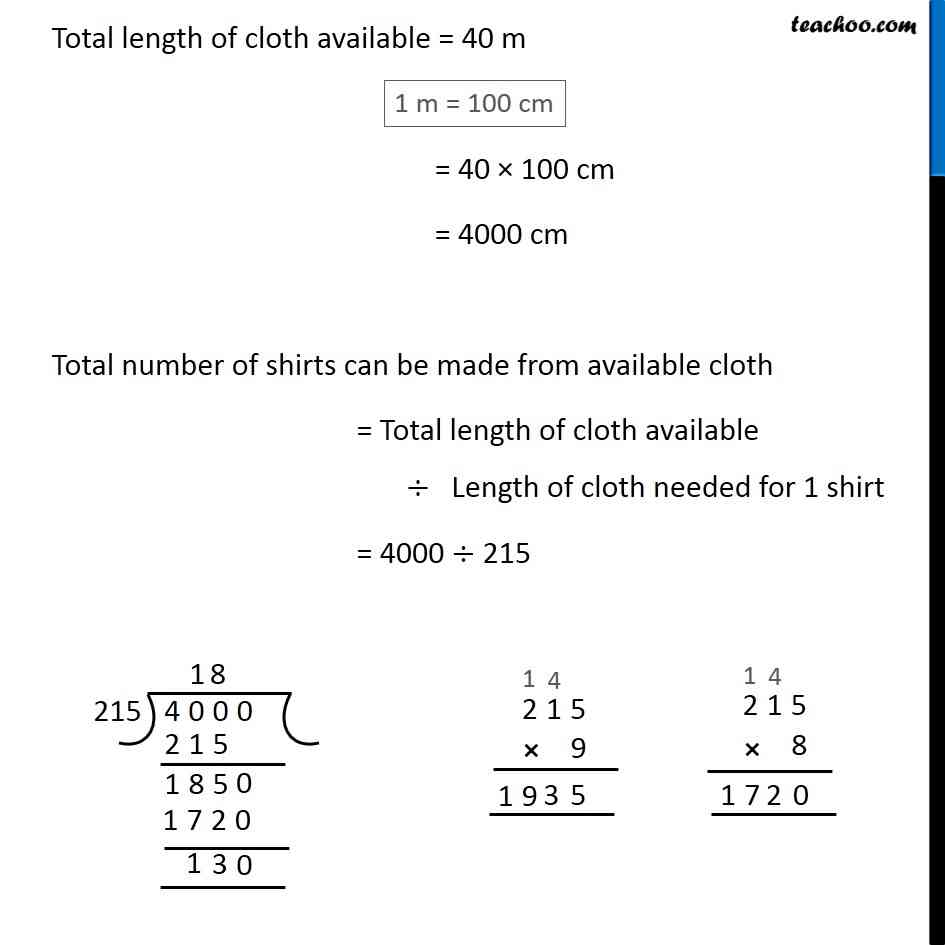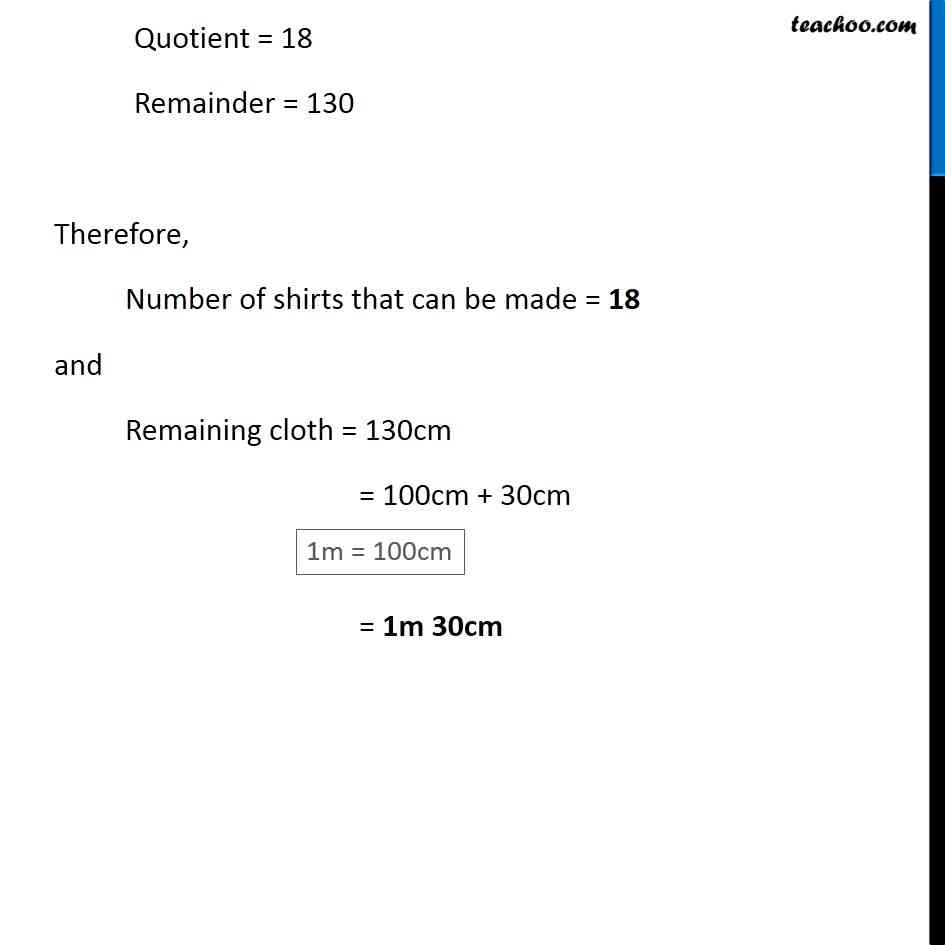Subscribe to our Youtube Channel - https://you.tube/teachoo

1. Chapter 1 Class 6 Knowing our Numbers
2. Concept wise
3. Division of large numbers

Transcript

Ex 1.2, 9 To stitch a shirt, 2 m 15 cm cloth is needed. Out of 40 m cloth, how many shirts can be stitched and how much cloth will remain?Length of cloth needed for stitching 1 Shirt = 2 m 15 cm = 2 m + 15 cm = 2 × 1 m + 15 cm = 2 × 100 cm + 15 cm = 200 cm + 15 cm = 215 cm Total length of cloth available = 40 m = 40 × 100 cm = 4000 cm Total number of shirts can be made from available cloth = Total length of cloth available ÷ Length of cloth needed for 1 shirt = 4000 ÷ 215 Quotient = 18 Remainder = 130 Therefore, Number of shirts that can be made = 18 and Remaining cloth = 130cm = 100cm + 30cm = 1m 30cm

Division of large numbers